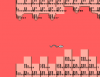# Urgent help, animation + positioning problem ...

M

#### Monopoxie

##### Guest
I have a problem with instance_position, it's returning noone when there are objects in coordinates, please help!There are 10 sprites in total and are set by what's next to it, see code below:

Code:
``````if x = (obj_sperm.x - 100) - (obj_control.view_wview) or del = true
{instance_destroy();}

r = instance_position(x+50,y,obj_wall);
if r = noone {instance_place(x+50,y,obj_wall);}
l = instance_position(x-50,y,obj_wall);
if l = noone {instance_place(x-50,y,obj_wall);}
u = instance_position(x,y+50,obj_wall);
if u = noone {instance_place(x,y+50,obj_wall);}
d = instance_position(x,y-50,obj_wall);
if d = noone {instance_place(x,y-50,obj_wall);}

if r > 000000
{right = true;}
if l > 000000
{left = true;}
if u > 000000
{up = true;}
if d > 000000
{down = true;}

if y < 300{
if right = true && left = true && up = true {

if u > 000000 {
if u.image_index = 6
{image_index = 0;}
if u.image_index = 4{
image_index = 7;}
if u.image_index = 2{
image_index = 8;}
if u.image_index = 3{
image_index = 9;}
}
}

if right = true && left = true && up = false {
image_index = 1;}
if right = true && left = false && up = false {
image_index = 2;}
if right = false && left = true && up = true {
image_index = 3;}
if right = false && left = false && up = false {
image_index = 4;}
if right = true && left = false && up = true {
image_index = 5;}
if right = false && left = true && up = true {
image_index = 6;}
}

if y > 300{
image_yscale = -1;

if right = true && left = true && down = true {

if d > 000000 {
if d.image_index = 6
{image_index = 0;}
if d.image_index = 4{
image_index = 7;}
if d.image_index = 2{
image_index = 8;}
if d.image_index = 3{
image_index = 9;}
}

}
if right = true && left = true && down = false {
image_index = 1;}
if right = true && left = false && down = false {
image_index = 2;}
if right = false && left = true && down = false {
image_index = 3;}
if right = false && left = false && down = false {
image_index = 4;}
if right = true && left = false && down = true {
image_index = 5;}
if right = false && left = true && down = true {
image_index = 6;}

if right = false && left = false && down = true {
image_index = 0;}

}``````Last edited by a moderator:
M

#### Monopoxie

##### Guest
I've tried multiple solutions, such as: assigning to grid, collision checking and calling IDs, this is the best result I got.

Last edited by a moderator:

#### Ting_Thing

##### Member
What's going on here?

Code:
``````r = instance_position(x+50,y,obj_wall);
if r = noone {instance_place(x+50,y,obj_wall);}
l = instance_position(x-50,y,obj_wall);
if l = noone {instance_place(x-50,y,obj_wall);}
u = instance_position(x,y+50,obj_wall);
if u = noone {instance_place(x,y+50,obj_wall);}
d = instance_position(x,y-50,obj_wall);
if d = noone {instance_place(x,y-50,obj_wall);}``````
You call instance_place for no apparent reason. instance_place doesn't do anything on its own. It's meant to give a value, but there is no variable assigned to the value it returns.

M

#### Monopoxie

##### Guest
Good spot, my bad but it still doesn't help the script. I'm going to simplify the sprite defining and see what I get.

Last edited by a moderator: